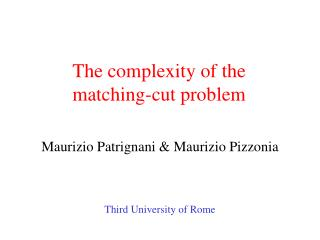# The complexity of the matching-cut problem - PowerPoint PPT PresentationDownload PresentationThe complexity of the matching-cut problem

The complexity of the matching-cut problemDownload Presentation## The complexity of the matching-cut problem

- - - - - - - - - - - - - - - - - - - - - - - - - - - E N D - - - - - - - - - - - - - - - - - - - - - - - - - - -
##### Presentation Transcript

1. The complexity of the matching-cut problem Maurizio Patrignani & Maurizio Pizzonia Third University of Rome

2. Overview • Application domain • Matching-cut problem • NAE3SAT reduction • Polynomial-time algorithm for series-parallel graphs • Conclusions

3. Three-dimensional orthogonal grid drawings of graphs A drawing of a K4 produced with the Interactive algorithm (Papakostas and Tollis 1997)

4. The “split & push” approach

5. End of the drawing process

6. A simpler example

7. “Fork”: two adjacent edges cut by the split A bad choice of the cuts

8. A result that is not so nice dummy node representing a bend final bend

9. Bad VS good cuts Reducing the number of edges cut by each split Reducing the forks produced by the cuts Details in: Di Battista, Patrignani, and Vargiu, "A Split&Push Approach to 3D Orthogonal Drawing", Journal of Graph Algorithms and Applications, 2000

10. The matching-cut problem A cut A matching A matching-cut Matching-Cut Problem Instance: A graph Question: Does a set of edges exist, such that it is a cut and a matching?

11. Previous work • Recognizing “decomposable graphs” is NP-complete even with graph of maximum degree 4, but it is polynomial for graphs of maximum degree 3 (V. Chvátal, 1984) • The problem remains NP-complete even restricting to bipartite graphs of minimum degree two (A.M. Moshi, 1989) • The problem remains NP-complete even restricting to bipartite graphs with one color class of nodes of degree 4 and the other color class of nodes of degree 3 (V.B. Le and B. Randerath, 2001)

12. x1 x3 x4 x2 x3 x4 x2 x3 x4 The NAE3SAT reduction Not-All-Equal-3-SAT Problem Instance: A set of clauses, each containing 3 literals from a set of boolean variables Question: Can truth values be assigned to the variables so that each caluse contains at least one true literal and at least one false literal? x1=false x2=true x3=true x4=true

13. Construction Observation: nodes joined by multiple edges can not be separated by a matching-cut false chain true chain

14. xi xi Variable gadget false chain true chain

15. xi xi xi xi Variable gadget matching-cuts false chain false chain false chain xi xi true chain true chain true chain xi is false (xi is true) xi is true (xi is false) Not allowed!

16. l m n Clause gadget For each clause false chain m n l true chain

17. m n l m n l m n l Clause gadget matching-cuts (1) l m n falsefalsetrue falsetruefalse falsetruetrue

18. m n l m n l m n l Clause gadget matching-cuts (2) l m n truefalsefalse truefalsetrue truetruefalse

19. x1 x3 x4 Connecting to variable gadgets Each node of the clause gadget that represents a literal is connected with two edges to the corresponding literal of the variable gadget Example: to x1 to x4 x3 x4 x3 x3 x1

20. x1 x2 x3 x2 x1 x3 x3 x3 x2 x2 x1 x1 An example of instance A NAE3SAT instance may be: The corresponding matching-cut instance is:

21. x1 x2 x3 x1 x3 x3 x2 x1 A solution x1=true x2=true x3=true A NAE3SAT solution to is: The corresponding matching-cut solution is: x2 x3 x2 x1

22. Graphs of maximum degree four Observation: each node of the construction has even degree replace each star with a “wheel”

23. Simple graphs Observation: multiple edges occur only in pairs replace each pair of edges with a triangle

24. Series-parallelgraphs A series-parallel graph has a source s and a sink t and can be constructed by recursively applying the following rules: Serial composition: starting from G1(s1,t1) and G2(s2,t2), obtain G(s1,t2) by identifying t1 and s2 s Basic step: a single edge between s and t is a series-parallel graph G(s,t) t s1 s1 = s2 t1 = s2 Parallel composition: starting from G1(s1,t1) and G2(s2,t2), obtain G(s1,t1) by identifying sources and sinks t2 t1 = t2

25. Parse tree construction A parse tree can be constructed in linear-time describing a sequence of operations producing the series-parallel graph. series edge parallel edge edge

26. s s label 1 label 1 false true t t Non st-separating matching-cuts We associate with each node of the parse tree two labels describing the properties of the intermediate series-parallel graph with respect to the existence of a matching-cut Label 1 signals if a non st-separating matching cut exists in the series-parallel graph

27. label 2 label 2 0 s St-separating matching-cuts Label 2 signals under which conditions the series-parallel graph admits an st-separating matching-cut s s s label 2 s AND t t t t s s s label 2 label 2 label 2 t s OR t 1 t t t

28. label 2 label 2 label 2 label 2 label 2 s AND t s AND t 0 s AND t s label 1 label 1 label 1 label 1 label 1 false false false false false Polynomial-time algorithm Traverse the parse tree top-down and update the labels. series edge parallel edge edge

29. Conclusions and open problems • We showed an interesting application domain for the matching-cut problem in the graph drawing field • We proved that the matching-cut problem is NP-complete by using a reduction of the NAE3SAT problem • The result can be extended to graphs of maximum degree four and to simple graphs • We produced a polynomial-time algorithm for series-parallel graphs • It is open whether the problem retains its complexity for planar graphs